Скачать презентацию Solving Equations STEPS 1 Locate Variable 2 Add or Subtract

3edb2acc7da1fcd7084c45782e4affe7.ppt

• Количество слайдов: 15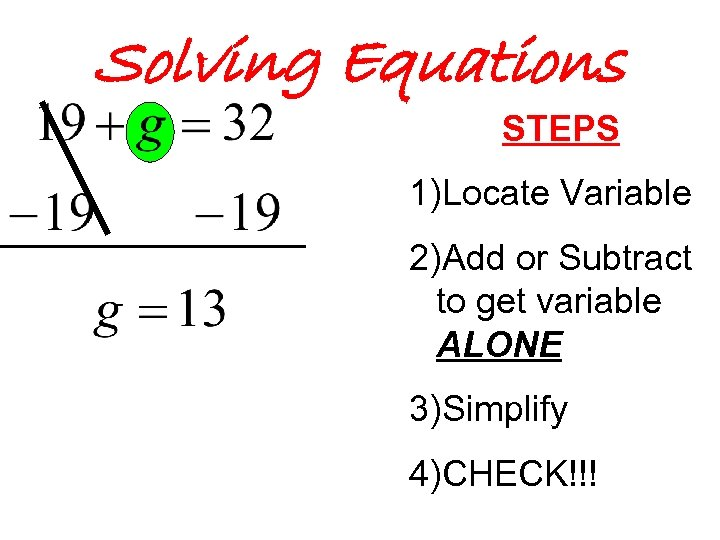Solving Equations STEPS 1)Locate Variable 2)Add or Subtract to get variable ALONE 3)Simplify 4)CHECK!!!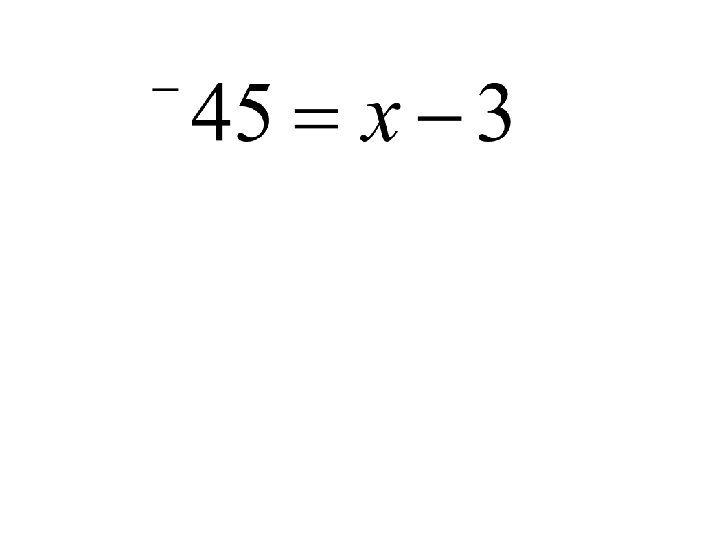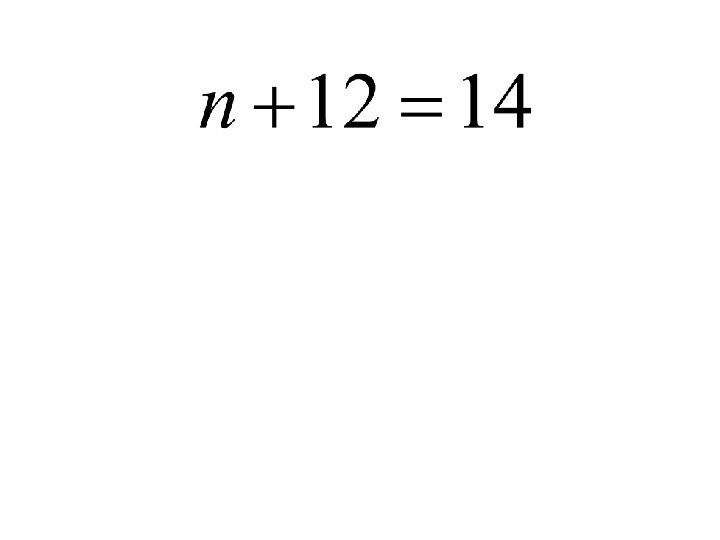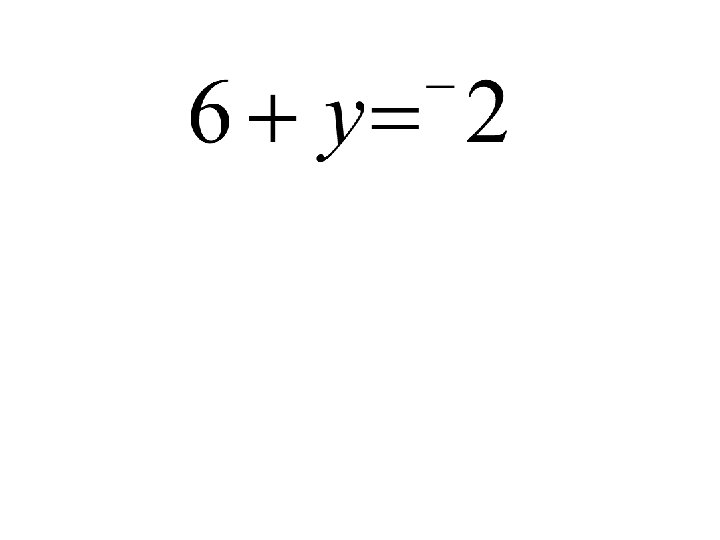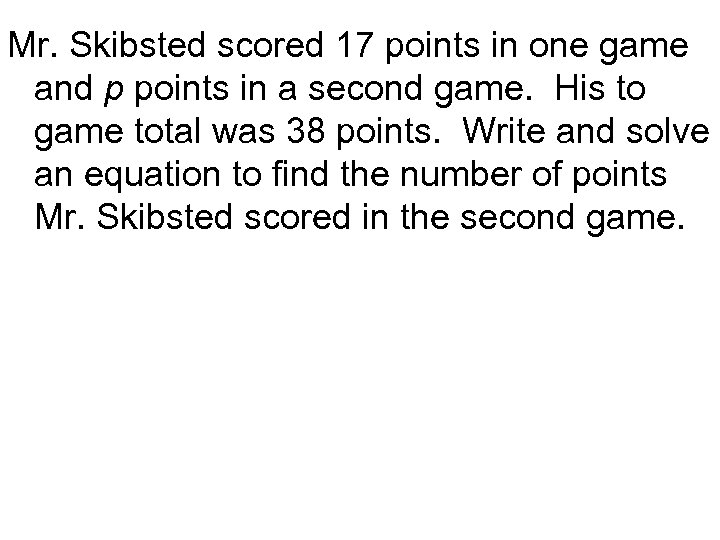Mr. Skibsted scored 17 points in one game and p points in a second game. His to game total was 38 points. Write and solve an equation to find the number of points Mr. Skibsted scored in the second game.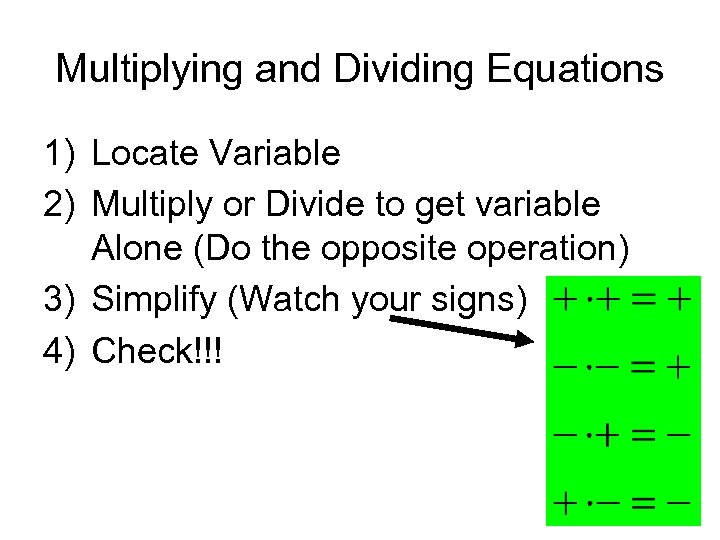Multiplying and Dividing Equations 1) Locate Variable 2) Multiply or Divide to get variable Alone (Do the opposite operation) 3) Simplify (Watch your signs) 4) Check!!!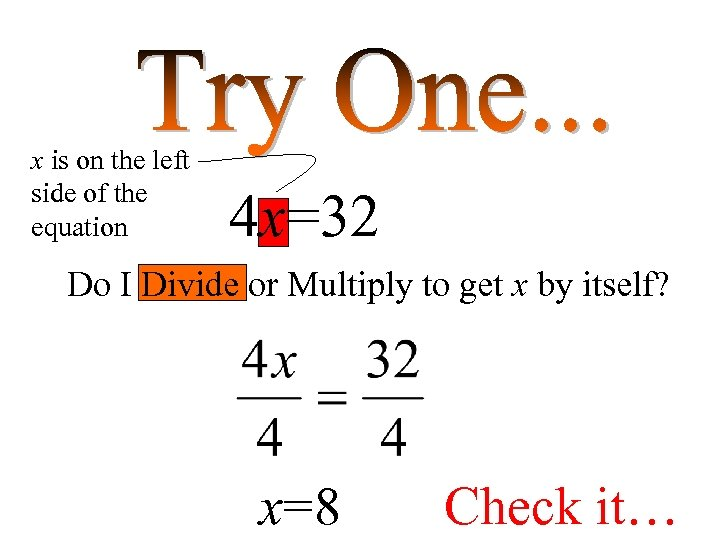x is on the left side of the equation 4 x=32 Do I Divide or Multiply to get x by itself? x=8 Check it…Check These Out…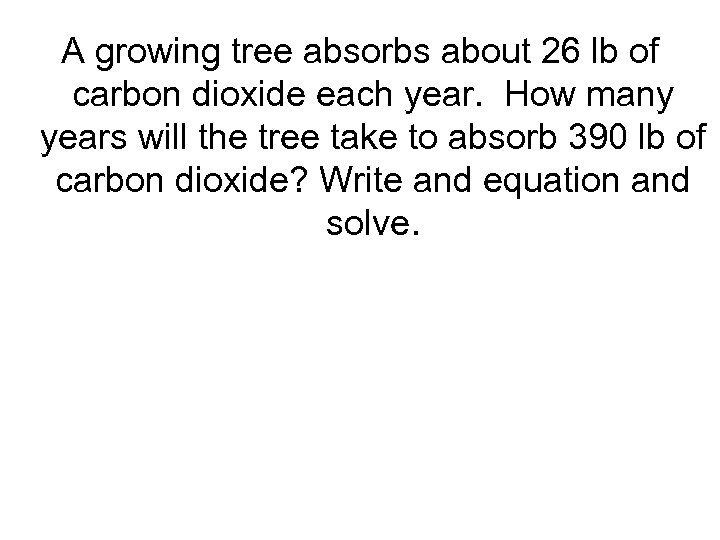A growing tree absorbs about 26 lb of carbon dioxide each year. How many years will the tree take to absorb 390 lb of carbon dioxide? Write and equation and solve.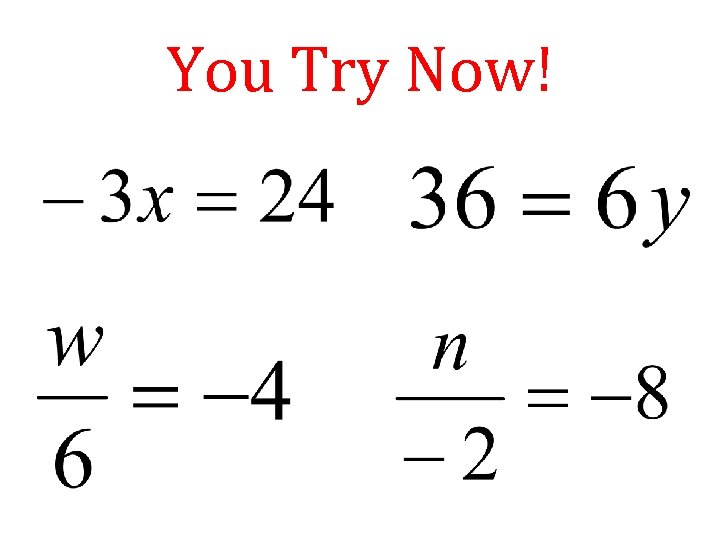You Try Now!Changing Units In The Customary System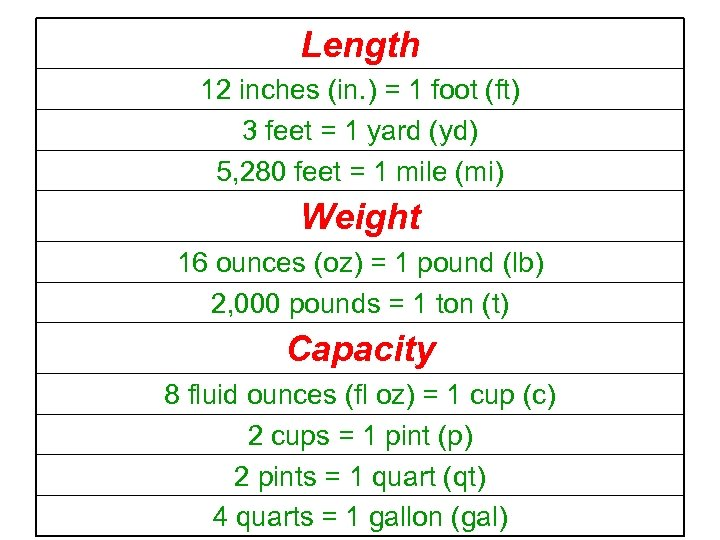Length 12 inches (in. ) = 1 foot (ft) 3 feet = 1 yard (yd) 5, 280 feet = 1 mile (mi) Weight 16 ounces (oz) = 1 pound (lb) 2, 000 pounds = 1 ton (t) Capacity 8 fluid ounces (fl oz) = 1 cup (c) 2 cups = 1 pint (p) 2 pints = 1 quart (qt) 4 quarts = 1 gallon (gal)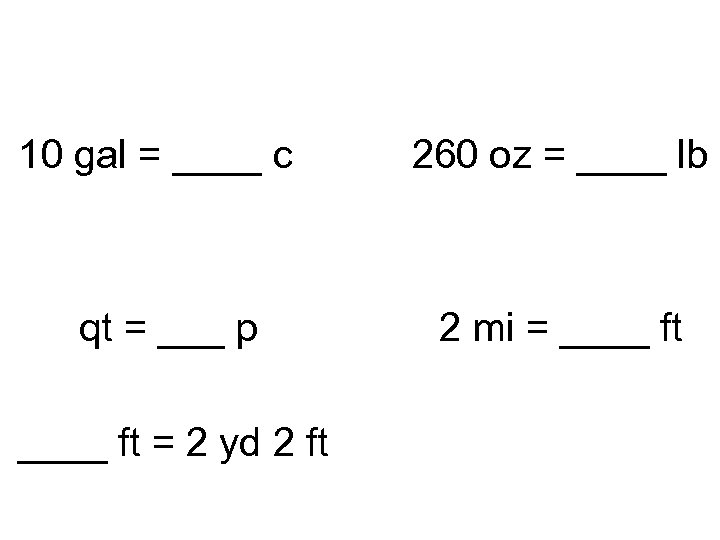10 gal = ____ c qt = ___ p ____ ft = 2 yd 2 ft 260 oz = ____ lb 2 mi = ____ ft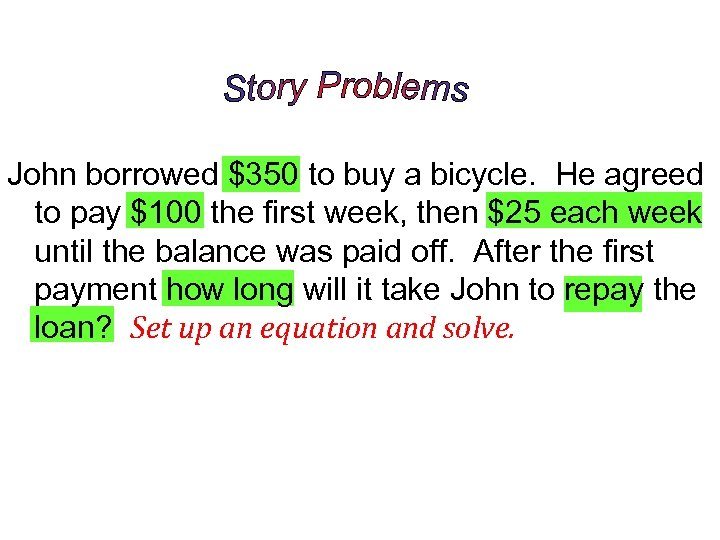John borrowed \$350 to buy a bicycle. He agreed to pay \$100 the first week, then \$25 each week until the balance was paid off. After the first payment how long will it take John to repay the loan? Set up an equation and solve.